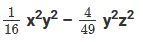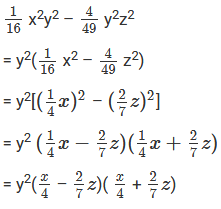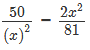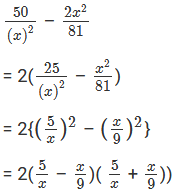×#### Thank you for registering.

One of our academic counsellors will contact you within 1 working day.

Click to Chat

1800-1023-196

+91-120-4616500

CART 0

• 0

MY CART (5)

Use Coupon: CART20 and get 20% off on all online Study Material

ITEM
DETAILS
MRP
DISCOUNT
FINAL PRICE
Total Price: Rs.

There are no items in this cart.
Continue Shopping• Complete JEE Main/Advanced Course and Test Series
• OFFERED PRICE: Rs. 15,900
• View Details

```Chapter 7: Factorization Exercise – 7.5

Question: 1

Solve:

16x2 – 25y2

Solution:

16x2 – 25y2

= (4x)2 – (5y)2

= (4x – 5y)(4x + 5y)

Question: 2

Solve:

27x2 – 12y2

Solution:

27x2 – 12y2

= 3(9x2 – 4y2)

= 3[(3x)2 – (2y)2]

= 3(3x – 2y)(3x + 2y)

Question: 3

Solve:

144a2 – 289b2

Solution:

144a2 – 289b2

= (12a)2 – (17b)2

= (12a – 17b)(12a + 17b)

Question: 4

Solve:

12m2 – 27

Solution:

12m2 – 27

= 3(4m2 – 9)

= 3[(2m)2 – 32]

= 3(2m – 3)(2m + 3)

Question: 5

Solve:

125x2 – 45y2

Solution:

125x2 – 45y2

= 5(25x2 – 9y2)

= 5[(5x)2 – (3y)2]

= 5(5x – 3y)(5x + 3y)

Question: 6

Solve:

144a2 – 169b2

Solution:

144a2 – 169b2

= (12a)2 – (13b2)

= (12a – 13b)(12a + 13b)

Question: 7

Solve:

(2a – b)2 – 16c2

Solution:

(2a – b)2 – 16c2

= (2a – b)2 – (4c)2

= [(2a – b) –  4c][(2a – b) + 4c]

= (2a – b – 4c)(2a – b + 4c)

Question: 8

Solve:

(x + 2y)2 – 4(2x – y)2

Solution:

(x + 2y)2 – 4(2x – y)2 = (x + 2y)2 – [2(2x – y)]2

= [(x + 2y) – 2(2x – y)][(x + 2y) + 2(2x – y)]

= (x + 2y – 4x + 2y)(x + 2y + 4x – 2y)

= 5x(4y – 3x)

Question: 9

Solve:

3a5 – 48a3

Solution:

3a5 – 48a3

= 3a3(a2 – 16)

= 3a3(a2 – 42)

= 3a3(a – 4)(a + 4)

Question: 10

Solve:

a4 – 16b4

Solution:

a4 – 16b4

= a4 – 24b4

= (a2)2 – (22b2)2

= (a2 – 22b2)(a2 + 22b2)

= [a2 – (2b)2](a2 + 4b2)

(a – 2b)(a + 2b)(a2 + 4b2)

Question: 11

Solve:

x8– 1

Solution:

x8– 1 = (x4)2 – 12

= (x4 – 1)(x4 + 1)

= [(x2)2 – 12](x4 + 1)

= (x2 – 1)(x2 + 1)(x4 + 1)

= (x2 – 12)(x2 + 1)(x4 + 1)

= (x – 1)(x +1)(x2 + 1)(x4 + 1)

Question: 12

Solve:

64 – (a + 1)2

Solution:

64 – (a + 1)2

= (8)2 – (a + 1)2

= [8 – (a + 1)][8 + (a + 1)]

= (8 – a – 1)(8 + a + 1)

= (7 – a) (9 + a)

Question: 13

Solve:

36L2 – (m + n)2

Solution:

36L2 – (m + n)2

= (6L)2 – (m + n)2

= [6L – (m +n)][6L + (m +n)]

= (6L – m – n)(6L + m + n)

Question: 14

Solve:

25x4y4 – 1

Solution:

25x4y4 – 1

= (5x2y2)2 – 1

= (5x2y2 – 1)(5x2y2 + 1)

Question: 15

Solve:

a4 – 1/b4

Solution:

a4 – 1/b4

= (a2)2 – 1/(b2)2

= a2 – 1/b2a2 + 1/b2

= a – 1/ba + 1/ba2 + 1/b2

Question: 16

Solve:

x3  – 144x

Solution:

x3  – 144x

= x(x2 – 144)

= x(x2 – 122)

= x(x – 12)(x + 12)

Question: 17

Solve:

(x – 4y)2 – 625

Solution:

(x – 4y)2 – 625

= (x – 4y)2 – 252

= [(x – 4y) – 25][(x – 4y) + 25]

= (x – 4y – 25)(x – 4y + 25)

Question: 18

Solve:

9(a – b)2 – 100(x – y)2

Solution:

9(a – b)2 – 100(x – y)2

= [3(a – b)]2 – [10(x – y)]2

= [3(a – b) – 10(x – y)][3(a – b) + 10(x – y)]

= (3a – 3b – 10x + 10y)(3a – 3b + 10x – 10y)

Question: 19

Solve:

(3 + 2a)2 – 25a2

Solution:

(3 + 2a)2 – 25a2

= (3 + 2a)2 – (5a)2

= [(3 + 2a) – 5a][(3 + 2a) + 5a]

= (3 + 2a – 5a)(3 + 2a + 5a)

= (3 – 3a)(3 + 7a)

= 3(1 - a)(3 + 7a)

Question: 20

Solve:

(x + y)2 – (a – b)2

Solution:

(x + y)2 – (a – b)2

= [(x + y) – (a – b)][(x + y) + (a – b)]

= (x + y – a + b)(x + y + a – b)

Question: 21

Solve:Solution:Question: 22

Solve:

75a3b2 – 108ab4

Solution:

75a3b2 – 108ab4

= 3ab2(25a2 – 36b2)

= 3ab2 [(5a)2 – (6b)2]

= 3ab2 (5a – 6b) (5a + 6b)

Question: 23

Solve:

x5 – 16x3

Solution:

x5 - 16x3

= x3(x2 – 16)

= x3(x2 – 42)

= x3(x – 4)(x + 4)

Question: 24

Solve:Solution:Question: 25

Solve:

256x5 – 81x

Solution:

256x5 – 81x

= x(256x4 – 81)

= x[(16x2)2 – 92]

= x(16x2 + 9)(16x2 – 9)

= x(16x2 + 9)[(4x)2 – 32]

= x(16x2 + 9)(4x + 3)(4x – 3)

Question: 26

Solve:

a4- (2b + c)4

Solution:

a4 – (2b + c)4

= (a2)2 – [(2b + c)2]2

= [a2 + (2b + c)2][a2 – (2b + c)2]

= [a2 + (2b + c)2]{[a + (2b + c)][a – (2b + c)]}

= [a2 + (2b + c)2](a + 2b + c)(a – 2b – c)

Question: 27

Solve:

(3x + 4y)4 – x4

Solution:

(3x + 4y)4 – x4

= [(3x + 4y)2]2 – (x2)2

= [(3x + 4y)2 + x2][(3x + 4y)2 – x2]

= [(3x + 4y)2 + x2][(3x + 4y) + x] [(3x + 4y) – x]

= {(3x + 4y)2 + x2} (3x + 4y + x)(3x + 4y – x)

= {(3x + 4y)2 + x2} (4x + 4y)(2x + 4y)

= {(3x + 4y)2 + x2} 4(x + y) 2 (x + 2y)

= 8{(3x + 4y)2 + x2} (x + y)(x + 2y)

Question: 28

Solve:

p2q2 – p4q4

Solution:

p2q2 – p4q4

= p2q2(1 – p2q2)

= p2q2 [1 – (pq)2]

= p2q2 (1 – pq)(1 + pq)

Question: 29

Solve:

3x3y – 243xy3

Solution:

3x3y – 243xy3

= 3xy(x2 – 81y2)

= 3xy[x2 – (9y)2]

= 3xy(x – 9y)(x + 9y)

Question: 30

Solve:

a4b4 – 16c4

Solution:

a4b4 – 16c4

= [(a2b2)2 – (4c2)2]

= (a2b2 + 4c2)(a2b2 – 4c2)

= (a2b2 + 4c2)[(ab)2 – (2c)2]

= (a2b2 + 4c2)(ab + 2c)(ab – 2c)

Question: 31

Solve:

x4 – 625

Solution:

x4 – 625

= (x2)2 – 252

= (x2 + 25)(x2 – 25)

= (x2+ 25)(x2 – 52)

= (x2 + 25)(x + 5)(x – 5)

Question: 32

Solve:

x4 – 1

Solution:

x4 – 1

= (x2)2 – 1

= (x2 + 1)(x2 – 1)

= (x2 + 1)(x + 1)(x – 1)

Question: 33

Solve:

49(a – b)2 – 25(a + b)2

Solution:

49(a – b)2 – 25(a + b)2

= [7(a – b)2] – [5(a + b)]2

= [7(a – b) – 5(a + b)] [7(a – b) + 5(a + b)]

= (7a – 7b – 5a – 5b)(7a – 7b + 5a + 5b)

= (2a – 12b)(12a – 2b)

= 2(a – 6b) 2(6a – b)

= 4(a – 6b)(6a – b)

Question: 34

Solve:

x – y – x2 + y2

Solution:

x – y – x2 + y2

= (x – y) + (y2 – x2)

= (x – y) + (y + x)(y – x)

= (x – y) – (y + x)(x – y)   [since, (y – x) = – (x – y)]

= (x – y)[1 – (y + x)]

= (x – y)(1 – x – y)

Question: 35

Solve:

16(2x – 1)2 – 25y2

Solution:

16(2x – 1)2 – 25y2

= [4(2x – 1)]2 – (5y)2

= [4(2x – 1) – 5y][4(2x – 1) + 5y]

= (8x – 4 – 5y)(8x -4 + 5y)

= (8x – 5y – 4)(8x + 5y – 4)

Question: 36

Solve:

4(xy + 1)2 – 9(x – 1)2

Solution:

4(xy + 1)2 – 9(x – 1)2

= [2(xy + 1)]2 – [3(x – 1)]2

= [2(xy + 1) – 3(x – 1)] [2(xy + 1) + 3(x – 1)]

= (2xy + 2 – 3x + 3)(2xy + 2 + 3x – 3)

= (2xy – 3x + 5)(2xy + 3x – 1)

Question: 37

Solve:

(2x + 1)2 – 9x4

Solution:

(2x + 1)2 – 9x4

= (2x + 1)2 – (3x2)2

= [(2x + 1) – 3x2][(2x + 1) + 3x2]

= (-3x2 + 2x + 1)(3x2 + 2x + 1)

= (-3x2 + 3x – x + 1)(3x2 + 2x + 1)

= {3x(-x + 1) + 1(-x + 1)}(3x2 + 2x + 1)

= (-x + 1)(3x + 1)(3x2 + 2x + 1)

= – (x – 1)(3x + 1)(3x2 + 2x + 1)

Question: 38

Solve:

x4 – (2y – 3z)2

Solution:

x4 – (2y – 3z)2

= (x2)2 – (2y – 3z)2

= [x2 – (2y – 3z)][x2 + (2y – 3z)]

= (x2 – 2y + 3z)(x2 + 2y – 3z)

Question: 39

Solve:

a2 – b2 + a – b

Solution:

a2 – b2 + a –b = (a2 – b2) + (a – b)

= (a + b)(a – b) + (a – b)

= (a – b)(a + b + 1)

Question: 40

Solve:

16a4 – b4

Solution:

16a4 – b4

= (4a2)2 – (b2)2

= (4a2 + b2)(4a2 – b2)

= (4a2 + b2)[(2a)2 – b2]

= (4a2 + b2)(2a + b)(2a – b)

Question: 41

Solve:

a4 – 16(b – c)4

Solution:

a4 – 16(b – c)4

= (a2)2 – [4(b – c)2]2

= [a2 + 4(b – c)2][a2 – 4(b – c)2]

= [a2 + 4(b – c)2] [a2 – [2(b – c)]2]

= [a2 + 4(b – c)2][a + 2(b – c)][a – 2(b – c)]

= [a2 + 4(b – c)2](a + 2b – 2c)(a – 2b + 2c)

Question: 42

Solve:

2a5 – 32a

Solution:

2a5 – 32a

= 2a(a4 – 16)

= 2a[(a2)2 – 42]

= 2a(a2 + 4)(a2 – 4)

= 2a(a2 + 4)(a2 – 22)

= 2a(a2 + 4)(a + 2)(a – 2)

= 2a(a – 2)(a + 2)(a2 + 4)

Question: 43

Solve:

a4b4 – 81c4

Solution:

a4b4 – 81c4

= (a2b2)2 – (9c2)2

= (a2b2 + 9c2)(a2b2 – 9c2)

= (a2b2 + 9c2)[(ab)2 – (3c)2]

= (a2b2+ 9c2)(ab + 3c)(ab – 3c)

Question: 44

Solve:

xy9 – yx9

Solution:

xy9 – yx9

= xy(y8 – x8)

= xy[(y4)2 – (x4)2]

= xy(y4 + x4)[(y2)2 – (x2)2]

= xy(y4 + x4)(y2 + x2)(y2 – x2)

=  xy(y4 + x4)(y2 + x2)(y + x)(y – x)

Question: 45

Solve:

x3 – x

Solution:

x3 – x = x(x2 – 1)

= x(x – 1)(x + 1)

Question: 46

Solve:

18a2x2 – 32

Solution:

18a2x2 – 32

= 2(9a2x2 – 16)

= 2[(3ax)2 – 42]

= 2(3ax – 4)(3ax + 4)
```### Course Features

• 728 Video Lectures
• Revision Notes
• Previous Year Papers
• Mind Map
• Study Planner
• NCERT Solutions
• Discussion Forum
• Test paper with Video Solution